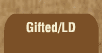Mathematics and Dyslexia

Tips for Learning the Times Tables

Tools for the Times Tables & Workbook

Home > Math > times tables practice > Big Nines in order

## The Nines Times Tables In Order - second set

The digits of the numbers that you can divide by nine always add up to nine. For 54, 5 + 4 is 9 etc. etc. One short cut to the nines tables answers is to go to one number less than what you're multiplying 9 by for the tens place, then put whatever adds up to nine in the ones place. It only sounds tricky. For 9 x 6 think "back one to 5...fifty...five and four is nine... fifty-four is the answer.

Or, just hold down your sixth finger (for 9 x 6)... count by tens on the fingers on the left, by ones on the fingers on the right [of course your thumbs count!!]... and there's your answer.

1. 9 x 6=
2. 9 x 7=
3. 9 x 8=
4. 9 x 9=
5. 9 x 10=
6. 9 x 10=
7. 9 x 9=
8. 9 x 8=
9. 9 x 7=
10. 9 x 6=
11. 6 x 9=
12. 9 x 7=
13. 8 x 9=
14. 9 x 9=
15. 10 x 9=